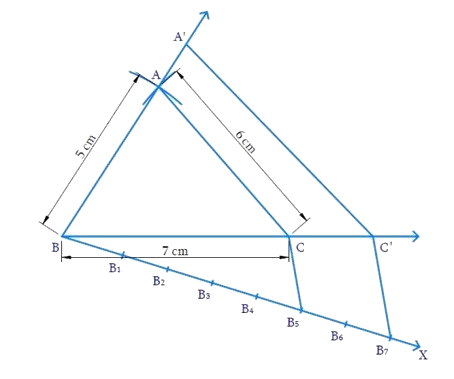# Ex.11.1 Q3 Constructions Solution - NCERT Maths Class 10

Go back to  'Ex.11.1'

## Question

Construct a triangle with sides $$5\, \rm{cm},$$ $$6\, \rm{cm}$$ and $$7 \,\rm{cm}$$ and then another triangle whose sides are \begin{align}\frac{7}{5}\end{align} of the corresponding sides of the first triangle.

Video Solution
Constructions
Ex 11.1 | Question 3

## Text Solution

#### What is known?

Sides of the triangle and the ratio of corresponding sides of $$2$$ triangles.

Construction.

#### Reasoning:

• Draw the line segment of largest length $$7 \,\rm{cm}$$. Measure $$5 \,\rm{cm}$$ and $$6 \,\rm{cm}$$ separately and cut arcs from $$2$$ ends of the line segment such that they cross each other at one point. Connect this point from both the ends.
• Then draw another line which makes an acute angle with the given line ($$7 \,\rm{cm}$$).
• Divide the line into $$m + n$$ parts where $$m$$ and $$n$$ are the ratio given.
• Two triangles are said to be similar if their corresponding angles are equal. They are said to satisfy Angle-Angle-Angle (AAA) Axiom.
• Basic proportionality theorem states that, “If a straight line is drawn parallel to a side of a triangle, then it divides the other two sides proportionally".

#### Steps:Steps of construction:

(i) Draw $${{BC = 7}}\,{\rm{cm}}$$ with $$B$$ and $$C$$ as centres and radii $$5\, \rm{cm}$$ and $$6 \,\rm{cm}$$ respectively. Draw arcs to intersect at $$A.$$ $$\,\Delta {{ABC}}$$ is obtained.

(ii) Draw ray $$BX$$ making $$\,\angle {{CBX}}$$ acute.

(iii) Mark $$7$$ points (greater of $$7$$ and $$5$$ in \begin{align}\frac{7}{5}\end{align} ) $${{{B}}_{{I}}}{{,}}\,{{{B}}_{{2}}}{{,}}\,{{ \ldots \ldots \ldots }}{{{B}}_{{7}}}$$ on $$BX$$ such that $$\,{{B}}{{{B}}_{{I}}}{{ = }}{{{B}}_{{I}}}{{{B}}_{{2}}}{{ = \ldots \ldots \ldots \ldots = }}{{{B}}_{{6}}}{{{B}}_{{7}}}$$

(iv) Join $${{{B}}_{{5}}}$$ (smaller of $$7$$ and $$5$$ in \begin{align}\frac{7}{5}\end{align} and so the $$5^\rm{th}$$ point) to $$C$$ and draw $${{{B}}_{{7}}}{{C'}}$$ parallel to $${{{B}}_{{5}}}{{C}}$$ intersecting the extension of $$BC$$ at  $$C'$$.

(v) Through $${{C'}}$$ draw $${{C'A'}}$$ parallel to $$CA$$ to meet the extension of $$BA$$ at $$A’.$$

Now, $$\,{{\Delta A'}}\,{{B'}}\,{{C'}}$$ is the required triangle similar to $$\,{{\Delta ABC}}$$ where

\begin{align}\frac{{{{BA'}}}}{{{{BA}}}}{{ = }}\frac{{{{C'A'}}}}{{{{CA}}}}{{ = }}\frac{{{{BC'}}}}{{{{BC}}}}{{ = }}\frac{{{7}}}{{{5}}}\end{align}

Proof:

In $$\Delta {B}{B}_{7}{C',}\;{B}_{3}{C}$$ is parallel to $${B}_{7}{C'}$$

Hence by Basic proportionality theorem,

\begin{align}\frac{{{{{B}}_{{6}}}{{{B}}_{{7}}}}}{{{{B}}{{{B}}_{{5}}}}}&{{ = }}\frac{{{{CC'}}}}{{{{BC}}}}\\&{{ = }}\frac{{{2}}}{{{5}}}\end{align}

Adding $$1$$,

\begin{align}\frac{{{{CC'}}}}{{{{BC}}}}{{ + 1}}& = \frac{{{2}}}{{{5}}}{{ + 1}}\\\frac{{{{BC + CC'}}}}{{{{BC}}}}{{ }}&=\frac{{{7}}}{{{5}}}\\\frac{{{{BC'}}}}{{{{BC}}}}{{ }}&= \frac{{{7}}}{{{5}}}\end{align}

Consider $${{\Delta BAC}}$$ and $${{\Delta BA'C'}}$$

$$\angle {{ABC}} = \angle {{A'BC'}}$$ (Common)

$$\angle \text{BCA}\!\!\text{ }=\angle \text{BC}\!'\text{A}\!\!'\!$$ (Corresponding angles $$∵$$ $$\text{CA}||\,\text{C }\!\!'\!\!\text{ }A'\$$ )

$$\angle {{BAC}} = \angle {{BA'C'}}$$ (Corresponding angles)

By AAA axiom, $$\Delta {{BAC}} \sim \Delta {{BA'C'}}$$

$$∴$$  Corresponding sides are proportional

Hence,

\begin{align}\frac{{{{BA'}}}}{{{{BA}}}}{{ = }}\frac{{{{C'A'}}}}{{{{CA}}}}{{ = }}\frac{{{{BC'}}}}{{{{BC}}}}{{ = }}\frac{{{7}}}{{{5}}}\end{align}

Learn from the best math teachers and top your exams

• Live one on one classroom and doubt clearing
• Practice worksheets in and after class for conceptual clarity
• Personalized curriculum to keep up with school